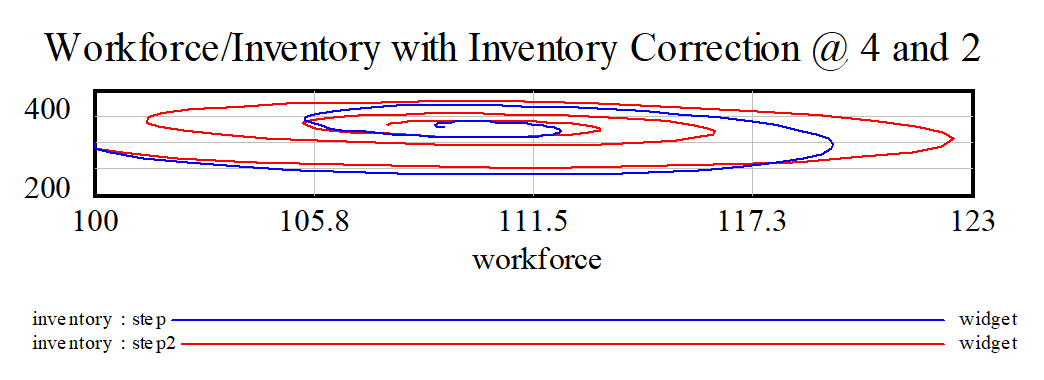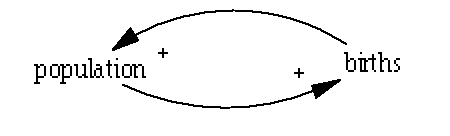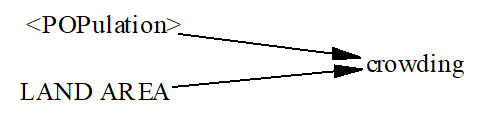# Vensim Help

 Queues

A Queue is simply a place where you put things and then take them away.  The most common example of a queue is a line that you have to wait in, and such a queue is called a FIFO queue which means first in first out (the first person to arrive is the first person served).  Vensim has some functions that make it easier to deal with keeping track of things in FIFO queues.

The QUEUE functions in Vensim all have two parts.  First these is a special level that, like a regular level, allows you to accumulate things, but keeping track of things by when they arrive and exit rather than lumping them together into one number.  For example suppose you are looking at order processing (:This is a traditional continuous approach to representing this and does not make use of any queues.  The equation for average cycle time is

average cycle time=ZIDZ(Work In Progress,shipped orders)

Compare this to a formulation using queues:The diagram is actually nearly the same, except for the Lookup called flat and the dropping of the arrow from shipped orders to average cycle time.  The changed equations are:

 Work In Progress= QUEUE FIFO( new orders, shipped orders,
 flat, 100, 3 )

Units: Widget

 shipped orders= MIN(processing capacity,
 QUEUE AGE IN RANGE(Work In Progress,
 minimum processing time, 1e+009)/TIME STEP)

Units: Widget/Day

average cycle time=QUEUE AGE AVERAGE(Work In Progress, 0)

Units: Day

If you compare simulations of these two models you will see that most variables behave the same with the exception of average cycle time.In the continuous formulation average cycle time increases from 2 to 32 in 30 days.  That could only be true if nothing were being produced and all the orders were place at time 20.  The formulation using QUEUE provides a more accurate answer.

In general the most value of the QUEUE functions comes because they give you the ability to get accurate cycle time and performance measurements.